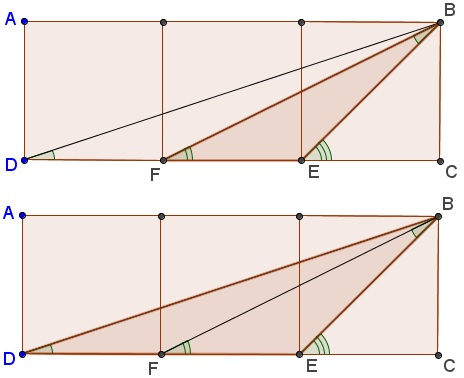# A Problem in Three Squares

### Explanation

An interesting fact holds in a configuration of three equal squares placed side by side. If the lines drawn as shown then $\angle BDC + \angle BFC = \angle BEC.\,$ There are many proofs of this result. These are so simple, the problem has been included in many a puzzle book.

First note that $\angle BEC = 45^{\circ}.\,$ The task is then to show that $\angle BDC + \angle BFC = 45^{\circ}.\,$ Let's introduce $\alpha = \angle BDC\,$ and $\beta = \angle BFC.\,$ We are to prove that $\alpha + \beta = 45^{\circ}.\,$

### Proof 1

Add another row of squares below the given three. $\Delta BKD\,$ is isosceles $(BK = DK)\,$ and, in addition, $\angle K = 90^{\circ}.\,$ Therefore, $\angle BDK = 45^{\circ}.\,$ However, since triangles $DEK\,$ and $FCB\,$ are equal, we also have $\angle KDE = \beta .$

### Proof 2

Now with some trigonometry $(\arctan = \tan^{-1}),$

\displaystyle \begin{align} \alpha + \beta &= \arctan(\frac{1}{3}) + \arctan(\frac{1}{2})\\ &= \arctan((\frac{1}{3} + \frac{1}{2})/(1 - \frac{1}{3}\cdot \frac{1}{2}))\\ &= \arctan(1). \end{align}

where I used the standard formula for the tangent of a sum

$\displaystyle \tan(\alpha + \beta ) = \frac{\tan\alpha + \tan\beta}{1 - \tan\alpha\cdot \tan\beta}.$

Since $\arctan(1) = 45^{\circ},\,$ $\alpha + \beta = 45^{\circ}.$

### Proof 3

Triangles $BDE\,$ and $FEB\,$ are similar.Indeed, assuming the side of the square equals $1,\,$ then

$\displaystyle \begin{array}{lll} \,DE=2, & BE=\sqrt{2}, &\displaystyle \frac{DE}{BE}=\sqrt{2},\\ BE=\sqrt{2}, & EF=1, &\displaystyle \frac{BE}{EF}=\sqrt{2},\\ BD=\sqrt{10}, & BF=\sqrt{5}, &\displaystyle \frac{BD}{BF}=\sqrt{2}. \end{array}$

which implies that $\angle BDC = \angle FBE = \alpha.\,$ Finally, the exterior angle $BEC\,$ of $\Delta FEB,\,$ equals the sum of the opposite interior angles, i.e., $\alpha + \beta.\,$ In short, we proved that

$\displaystyle \arctan(\frac{1}{2}) + \arctan(\frac{1}{3}) = \arctan(1).$

Observe that this implies another identity:

$\displaystyle \arctan(1) + \arctan(\frac{1}{2}) + \arctan(\frac{1}{3}) = \frac{\pi}{2}.$

### Proof 4

Following a proof without words by Hasan Unal (Math Magazine, v 82, n 1, February 2009 , p. 56) we shall prove a more general statement. Let x and y be positive numbers satisfying $x^{2} + y^{2} = 1.\,$ Then

$\displaystyle\arctan\frac{1-x}{y}+\arctan\frac{1-y}{x}=\frac{\pi}{4}.$

### TrigonometryCopyright © 1996-2018 Alexander Bogomolny

 65268610